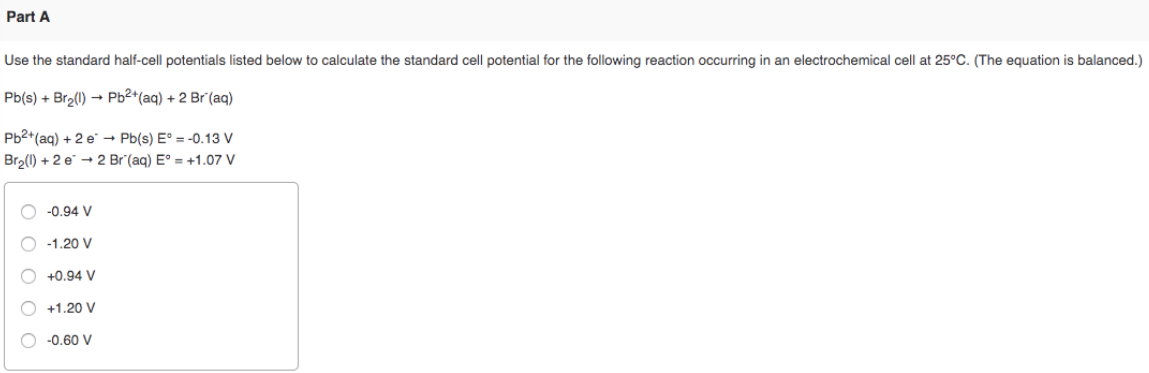# Use the standard half-cell potentials listed below to calculate the standard cell potential for the following reaction occurring in an electrochemical cell at 25°C. (The equation is balanced.) Pb(s) + Br2(l) → Pb^2+(aq) + 2 Br^-(aq) Pb^2+(aq) + 2 e^- → Pb(s) E° = -0.13 V Br2(l) + 2 e^- → 2 Br^-(aq) E° = +1.07 V a) -0.94 V b) -1.20 V c) +0.94 V d) +1.20 V e) -0.60 V